# GMAT Math : Calculating the length of an edge of a prism

## Example Questions

### Example Question #1 : Calculating The Length Of An Edge Of A Prism

A new fish tank at a theme park must hold 450,000 gallons of sea water. Its dimensions must be such that it is twice as long as it is wide, and half as high as it is wide. If one gallon of water occupies 0.1337 cubic feet, then give the surface area of the proposed tank to the nearest square foot.

You may assume that the tank has all four sides and a bottom, but is open at the top.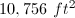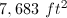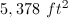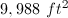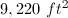Explanation:

450,000 gallons of water occupy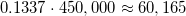cubic feet.

Letbe the height of the tank. Then the width of the tank is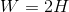, and its length is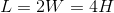. Multiply the dimensions to get the volume: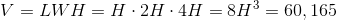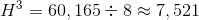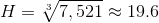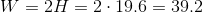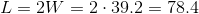Since the tank has four sides and a bottom, but not a top, its surface area is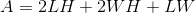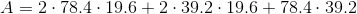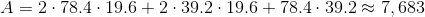The surface area of the tank is about 7,683 square feet.

### Example Question #2 : Calculating The Length Of An Edge Of A Prism

A rectangular prism has a volume of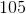.  If the length of the prism isand its width is, what is its height?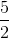Explanation:

The volume of a rectangular prism is equal to its length times its width times its height. We are given the volume, the length, and the width, so using the following formula we can solve for the height of the rectangular prism: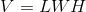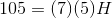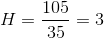### Example Question #3 : Calculating The Length Of An Edge Of A Prism

A rectangular prism has a volume of,  a length of,  and a height of.  What is the width of the prism?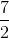Explanation:

Using the formula for the volume of a rectangular prism, we can plug in the given values and solve for the width of the prism: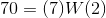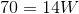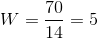### Example Question #4 : Calculating The Length Of An Edge Of A Prism

A rectangular prism has a surface area of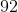,  a width of,  and a height of.  What is the length of the prism?Explanation:

We are given the surface area and the length of two sides, so in order to calculate the length of the third side we need the formula for the surface area of a prism in terms of each side length: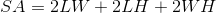Using the formula, we can simply plug in the given values and solve for the length of the rectangular prism: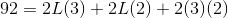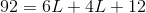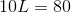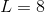### Example Question #5 : Calculating The Length Of An Edge Of A Prism

Jenny wants to make a cube out of sheet metal. What is the length of one side of the cube?

I) The cube will require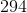square inches of material.

II) The cube will hold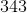cubic inches.

Statement I is sufficient to answer the question, but statement II is not sufficient to answer the question.

Both statements are needed to answer the question.

Either statement is sufficient to answer the question.

Statement II is sufficient to answer the question, but statement I is not sufficient to answer the question.

Either statement is sufficient to answer the question.

Explanation:

The length of an edge of a cube can be used to find either volume or surface area, and vice versa.

I) Gives us the surface area thus, we are able to calculate the length of an edge using the formula,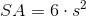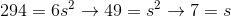.

II) Gives us the volume thus, we are able to calculate the length of an edge using the formula,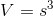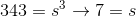Either can be used to find the side length.

Tired of practice problems?

Try live online GMAT prep today.